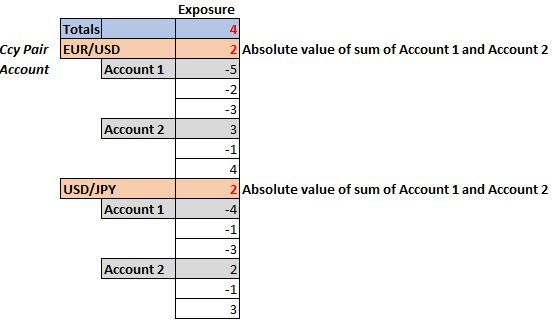# Qlik Sense App Development

Announcements
BI & Data Trends 2021. Discover the top 10 trends emerging in today. Join us on Dec. 8th REGISTER
cancel
Showing results for
Did you mean:
HighlightedPartner

## Absolute sum at the dimension level in a pivot table in Qlik Sense

Hi,

I'm totally new to Qlik Sense. I have a pivot table with 2 dimensions: Ccy Pair and Account. I'm making a pivot table where the values have signs, but I want the sum at the Account level to be absolute value (please see the screenshot below). Is there any settings or function that can serve this purpose?4 Replies
HighlightedMVP

Assuming sum(Amount) is your measure.  You can replace with your actual measure

=if( Dimensionality()=1,
fabs(sum(aggr(Sum(Amount),Ccy_pair))),Sum(Amount))

HighlightedPartner

Hi Kush,

Thank you! I got absolute total at the Ccy Pair level, which is great! But the Totals  still show signed (negative) numbers. Is there any way to make Totals = sum of all absolute totals of Ccy Pairs?HighlightedPartner

Hello Lucy,

I wonder did you get the answer to the Total value? How could it also be shown in absolut value?

Thank you.

Lan

HighlightedMVP

@LucyN  try below

=if( Dimensionality()=1,
fabs(sum(aggr(Sum(Amount),Ccy_pair))),

if( Dimensionality()=0,
sum(aggr(fabs(sum(aggr(Sum(Amount),Ccy_pair))),Ccy_pair)),

Sum(Amount)))